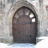Choose Language Hide Translation Bar

## Change Control Chart Size (Aspect Ratio)

I copy control charts from JMP and paste to Word documents. In Jmp 12 and before the charts had a narrow height and wide width that was condusive to pasting two charts on each page. In JMP 13 the chart is much more square and does not use the width of the Word page efficiently. I am attaching two versions of the control charts for viewing. I would like help returning JMP 13 to the control chart aspect ratio of JMP 12.

1 ACCEPTED SOLUTION

Accepted Solutionssusan_walsh1
Staff (Retired)

## Re: Change Control Chart Size (Aspect Ratio)

The examples that have been provided thus far are for the traditional control chart platform. It appears you are using Control Chart Builder which has a somewhat easier method of controlling the size of the graph. At the beginning of your script try this:

``````Control Chart Builder(
Size( 600, 450 ),
Variables( Y( ....``````

The first number in the Size statement controls the width of the graph; the second number controls the height of the graph.

You can try varying these values to get the size graph you need.

9 REPLIES 9

## Re: Change Control Chart Size (Aspect Ratio)

You can re-size the charts in the JMP report by dragging an edge of the chart.  Then save the script.  You can then reuse the script to produce the charts with the exact dimensions you like for pasting into MS Word.  I hope that helps.

## Re: Change Control Chart Size (Aspect Ratio)

I think you are on the right track. I can drag the edge to make the chart size better fit my needs but I can't figure out how to save that as part of the script. I use a script to generate the control charts. Are you suggesting I can modify those scripts to make the chart size change?

## Re: Change Control Chart Size (Aspect Ratio)

Here is an example of what you want.  I took the example from

Help==>Scripting Index==>Framebox

and switched out a control chart for their example, which was a Fit Y by X

``````Names Default To Here( 1 );
Open( "\$SAMPLE_DATA/Big Class.jmp" );
biv = Control Chart(
Sample Size( 5 ),
KSigma( 3 ),
Chart Col( :height, XBar, R )
);
rbiv = biv << report;
framebox = rbiv[Frame Box( 1 )];
framebox << Frame Size( 800, 300 );``````

Jim

## Re: Change Control Chart Size (Aspect Ratio)

Yes, re-sizing the chart and saving the script should generate the code you need for setting the size of the frame box.  Just copy and paste the extra snippets of code to your existing script.  For example I took the sample data table  "Braces" that alrady has script embeded to produce a U-chart.  I ran the script and then re-sized the chart and save the modified report as a new script.  Below I've include a copy of both scritps for comparison.  What I colored in red is the new code generated from re-sizing the chart, green was original.

Original Script copied to the sample data table:

``````Control Chart(
Sample Label( :Date ),
Unit Size( :Unit size ),
K Sigma( 3 ),
Chart Col( Name( "# defects" ), U )
)``````

New script that I saved to the data table after modifying the chart dimentions:

``````Control Chart(
Sample Label( :Date ),
Unit Size( :Unit size ),
KSigma( 3 ),
Chart Col( :Name( "# defects" ), U ),
SendToReport(
Dispatch(
{"U of # defects"},
"Attributes Chart",
FrameBox,
{Frame Size( 354, 193 )}
),
Dispatch(
{"U of # defects"},
"Attributes Chart",
FrameBox( 2 ),
{Frame Size( 55, 193 )}
)
)
)``````

## Re: Change Control Chart Size (Aspect Ratio)

I am still struggling with this. I am not much of a scripter. Here is my Control Chart Builder script. I have tried the sendtoreport and dispatch functions to no avail. I appreciate your attempts to help me with this.

``````Control Chart Builder(
Variables(
Y(
:Name( "% Oversize" ),
:Ms Temp.,
:Ideal Diameter,
:Name( "Grain Size, Surface" ),
:Name( "Grain Size, Center" ),
:Name( "C, LECO" ),
:Mn,
:P,
:S,
:Si,
:Ni,
:Cr,
:Mo,
:Cu,
:Ti,
:V,
:Nb,
:Al,
:Surface Hardness,
:Avg. Volumetric Hardness,
)
),
Chart( Position( 1 ) ),
Chart( Position( 2 ) ),

Where( :Source == "SINO" )
)``````susan_walsh1
Staff (Retired)

## Re: Change Control Chart Size (Aspect Ratio)

The examples that have been provided thus far are for the traditional control chart platform. It appears you are using Control Chart Builder which has a somewhat easier method of controlling the size of the graph. At the beginning of your script try this:

``````Control Chart Builder(
Size( 600, 450 ),
Variables( Y( ....``````

The first number in the Size statement controls the width of the graph; the second number controls the height of the graph.

You can try varying these values to get the size graph you need.

## Re: Change Control Chart Size (Aspect Ratio)

Perfect! Thank you!

## Re: Change Control Chart Size (Aspect Ratio)

I would like to be able to script the size of the data box, not the size of the window. How can this be done using graph builder?

Why?: When you make figures with many paralell data boxes but with different axes, the Size(x, y) does not help as the data frame is scaled to fit the text of the axis into the set window size. This way, when I align the figure panes in intaglio/illustrator, the data frames are of different sizes.

Many Thanks

## Re: Change Control Chart Size (Aspect Ratio)

In the Graph Preferences, it appears that for both JMP 12 and 13, the Height of graphs is set to 240. Maybe you could reduce that, and see if JMP will keep the width the same.
Jim